# Elasticity of Supply: Types, Methods and Factors

ADVERTISEMENTS:

The law of supply states the direct relationship between the price of a product and quantity supplied of the product.

In simple words, if the price of a product increases, the quantity supplied for the product also increases.

On the other hand, if there is fall in the price of a product, then the quantity supplied of the product would also decrease.

“The supply of a commodity is said to be elastic when as a result of a charge in price, the supply changes sufficiently as a quick response. Contrarily, if there is no change or negligible change in supply or supply pays no response, it is elastic”-Prof Thomas.

ADVERTISEMENTS:

However, it is not a quantitative statement. In practical implications, an organization needs to estimate the degree of change in the quantity supplied of a product with respect to change in the price of the product. The degree or extent of change in the quantity supplied of a product in response to change in the price of the product is known as the elasticity of supply.

According to Prof Thomas, “The supply of a commodity is said to be elastic when as a result of a charge in price, the supply changes sufficiently as a quick response. Contrarily, if there is no change or negligible change in supply or supply pays no response, it is elastic.” It can be calculated by dividing the percentage change in the quantity supplied with percentage change in the price of a product.

The formula for calculating elasticity of supply (eS) is as follows:

eS = Percentage change in quantity supplied/Percentage change in price

ADVERTISEMENTS:

Percentage change in quantity supplied = New quantity supplied (∆S)/Original quantity supplied (S)

Percentage change in price = New price (∆P)/Original Price (P)

The symbolic representation of elasticity of supply is as follows:

eS = ∆S/S : ∆P/P

ADVERTISEMENTS:

eS = ∆S/S * P/∆P

eS = ∆S/∆P * P/S

Change in quantity supplied (∆S) is the difference between the new quantity supplied (S1) and original quantity supplied(S).

It can be calculated by the following formula:

∆S = S1 – S

Similarly, change in price is the difference between the new price (P1) and original price (P).

It can be calculated by the following formula:

∆P = P1 – P

#### Types of Elasticity of Supply:

The degree of change in the quantity supplied with respect to change in the price of a product varies in different situations.

ADVERTISEMENTS:

Following are different types of elasticity of supply:

i. Perfectly Elastic Supply:

Refers to a situation when the quantity supplied completely increases or decreases with respect to proportionate change in the price of a product. In such a case, the numerical value of elasticity of supply ranges from zero to infinity (eS = 00)This situation is imaginary as there is no as such product whose supply is perfectly elastic.

Therefore the situation does not have any practical implication. In such a case, the price remains constant as the price of a product does not affect the quantity supplied. Let us understand the concept of perfectly elastic demand with the help of an example.

ADVERTISEMENTS: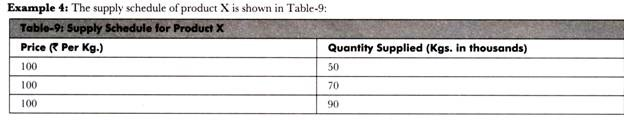Prepare a supply curve for the supply schedule of product X and determine the type of elasticity of supply demonstrated by the supply curve.

Solution:

The supply curve for product X is shown in Figure-15:

ADVERTISEMENTS: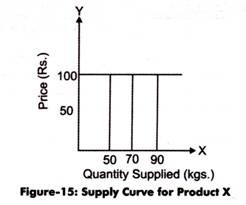Figure-15 shows that the price of product X remains constant at Rs. 100 per kg. However, the quantity supplied changes from 50,000 Kgs to 90,000 Kgs at the same price rate. Therefore, the supply of product X is perfectly elastic (eS = 00).

ii. Relatively Elastic Supply:

Refers to a condition when the proportionate change in the quantity supplied is more than proportionate change in the price of a product. In such a case, the numerical value of elasticity of supply is greater than one (eS>1) For example, if the quantity supplied increases by 30% with respect to 10% change in the price of a product, it is called relatively elastic supply. The concept of relatively elastic supply is explained with the help of an example.

Example 5:

The quantity supplied and the price of product P is shown in Table-10:

ADVERTISEMENTS:

Prepare a supply curve for the supply schedule of product P and determine the type of elasticity of supply demonstrated by the supply curve.

Solution:

The supply curve for product P is shown in Figure-16: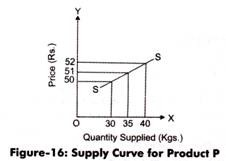In Figure-16, when the price of product P is Rs. 50, the quantity supplied is 30,000 Kgs. However, when the price increases to Rs. 51, supply reaches to 35,000. Similarly, when the price further increases to Rs. 52, the supply reduces to 40,000 Kgs.

ADVERTISEMENTS:

This shows that the change in price is only one rupee while the change in supply is 5,000. In other words, the proportionate change in quantity supplied is more than the proportionate change in the price of product P. Therefore, the supply of product P is highly elastic (eS>1).

iii. Relatively Inelastic Supply:

Refers to a condition when the proportionate change in the quantity supplied is less than proportionate change in the price of a product. In such a case, the numerical value of elasticity of supply is less than one (eS<1). For instance, the elasticity of supply would be less than unit, if the quantity supplied increases by 20% with respect to 30% change in the price of a product.

Example-6:

The quantity supplied and the price of product Z is shown in Table-11:

Prepare a supply curve for the supply schedule of product Z and determine the type of elasticity of supply demonstrated by the supply curve.

ADVERTISEMENTS:

Solution:

The supply curve for product Z is shown in Figure-17: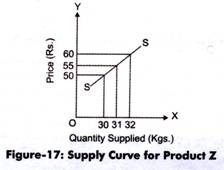In Figure-17, when the price of product Z is Rs. 50, the quantity supplied is 30,000 Kgs. When price increases to Rs. 55, supply reaches to 31, 000. Similarly, when the price of product Z increases to Rs. 60, the supply increases to 32,000 Kgs. This shows that S change in price is five rupees while the change in supply is 1,000. In other words, the proportionate change in quantity supplied is less than the change in the price of product Z. Therefore, the supply of product Z is relatively inelastic (eS<1).

iv. Unit Elastic Supply:

ADVERTISEMENTS:

Refers to a situation when the proportionate change in the quantity supplied is equal to the

Proportionate change in the price of a product. The numerical value of unit elastic supply is equal to one (eS=1).

Example 7: The quantity supplied and the price of product Y is shown in Table-12: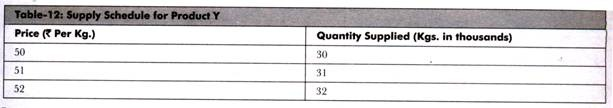Prepare a supply curve for the supply schedule of product Y and determine the type of elasticity of supply demonstrated by the supply curve.

Solution:

The supply curve for product Y is shown in Figure-18: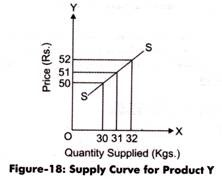In Figure-18, when the price of product Y is Rs. 50, the quantity supplied is 30,000 Kgs. When price increases to Rs. 51, supply reaches to 31,000. Similarly, when the price increases to Rs. 52, the supply increases to 32,000 Kgs. This shows that the proportionate change in quantity supplied is equal to the change in the price of product Y. Therefore, the supply of product Y is unit elastic (eS=1).

v. Perfectly Inelastic Supply:

Refers to a situation when the quantity supplied does not change with respect to proportionate change in price of a product. In such a case, the quantity supplied remains constant in all the instances of change in price. The numerical value of elasticity of supply is equal to zero. This situation is imaginary as there is no as such product whose

Supply is perfectly inelastic. Therefore, this situation does not have any practical implication.

Example 8:

The quantity supplied and the price of product R is shown in Table-13:

Prepare a supply curve for the supply schedule of product R and determine the type of elasticity of supply demonstrated by the supply curve.

Solution:

The supply curve for product R is shown in Figure-19: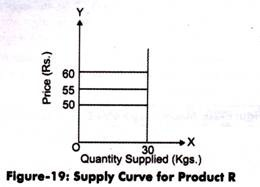Figure-19 shows that the supply of product R remains constant at 30,000 Kgs. However, the price changes from Rs. 50 to Rs. 60 at the same supply rate. Therefore, the supply of product X is perfectly inelastic (e = 0).

#### Methods of Measuring Elasticity of Supply:

Apart from determining the elasticity or inelasticity of supply, an organization needs to estimate the numerical value of elasticity of supply for making various business decisions.

The numerical value of elasticity of supply can be measured with the help of the following methods:

i. Proportionate Method:

Refers to one of the important methods of measuring elasticity of supply. In this method, elasticity of supply can be calculated by dividing the percentage change in quantity supplied with the percentage change in price of a product.

The formula used for calculating elasticity of supply through proportionate method is as follows:

eS = Percentage change in quantity supplied/Percentage change in price

Percentage change in quantity supplied = New quantity supplied (∆S)/Original quantity supplied (S)

Percentage change in price = New price (∆P)/original Price (P)

The symbolic representation of elasticity of supply is as follows:

eS = ∆S/S : ∆P/P

eS = ∆S/S * P/∆P

eS = ∆S/∆P * P/S

Change in quantity supplied (∆S) is the difference between the new quantity supplied (S) and original quantity supplied(S).

It can be calculated by the following formula:

∆S = S1-S

Similarly, change in price is the difference between the new price (P) and original price (P).

It can be calculated by the following formula:

∆P = P1 – P

For example, quantity supplied of a product increases from 1000 units to 1500 units and price changes from Rs. 50 to Rs. 55 per unit.

In such a case, the elasticity of supply would be as follows:

P1 = Rs. 55, P = Rs. 50, S1 = 1500 units, S = 1000 units

Therefore, ∆S = S1 – S = 1500 – 1000 = 500 units and ∆P = P1 – P = Rs. 55 – Rs. 50 = Rs. 5

eS = 500/5 * 50/1000 = 0.5 (less than unit)

According to this method, if the numerical value of elasticity of supply is more than one, it represents relatively elastic supply. On the other hand, if the numerical value of elasticity of supply is less than one, then the elasticity of supply would be relatively inelastic. Apart from this, if the numerical value of elasticity of supply is equal to one, it would represent unitary elastic supply.

ii. Point Method:

Refers to the method in which elasticity of supply is measured at a particular point on the supply curve. In such a case, to measure the elasticity of supply, a tangent needs to be drawn along with the demand curve.

The calculation of elasticity of supply at a particular point would be clearer with the help of Figure-20: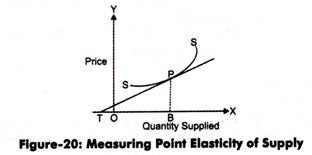In Figure-20, TF tangent is drawn from the point P to measure elasticity of supply. This tangent meets X axis at T point. Another vertical line from P is intersecting X-axis at B point.

Now, we can calculate elasticity of supply at point P as under:

eS = TB/OB

#### Factors Determining Elasticity of Supply:

The numerical value of elasticity of supply is different for different situations. The elasticity of supply is influenced by a number of factors.

Some of the factors that determine the elasticity of supply are as follows:

i. Nature of a Good:

Acts as a major determinant that influence the elasticity of supply. Goods, such as antiques and old wines, cannot be reproduced in the same form; therefore, the supply of such goods remains constant. Similarly, in case of perishable goods such as vegetables, fruits, and other eatables, the supply would be inelastic.

This is because the supply of perishable goods cannot be increased or decreased easily. On the contrary, in case of durable goods, such as furniture and electric appliances, the supply would be elastic as their supply can be increased or decreased quickly.

ii. Production Technology:

Refers to the level of technology that helps in determining the elasticity of supply. The supply of a good produced by using higher level technology is faster with respect to the change in its price.

iii. Time Period:

Affects the elasticity of supply to a larger extent. In short-run, elasticity of supply is low while in the long run elasticity of supply is more. Therefore, changes in prices do not affect the supply of a good immediately. If the price remains high for a longer period, only then suppliers prefer to increase the supply of product.

iv. Scale of Production:

Puts a significant impact on the elasticity of supply. In case of small-scale production of goods, the supply would be inelastic and vice versa. For example, if an organization has a large scale production of soaps, then an increase in the price of soaps would increase the supply of soaps without any time lag.

v. Agricultural Products:

Act as a major determinant of elasticity of supply in case of agricultural products. The supply of agriculture products, such as fruits, vegetables, and food grains, depends on natural factors, including ram, humidity, and sunlight. Therefore, the production of agricultural products cannot be increased or decreased easily. Consequently, the supply of these products is relatively inelastic.